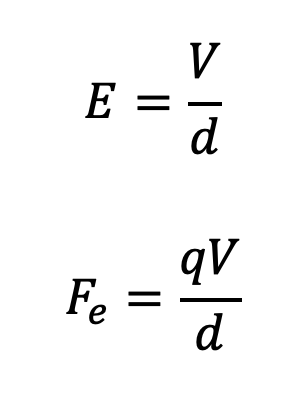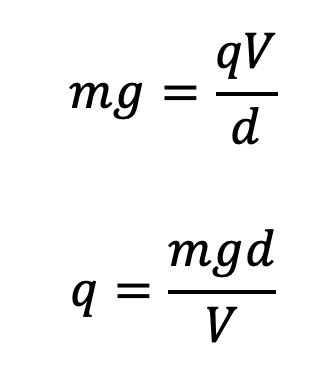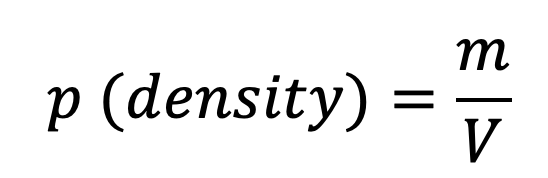# Millikan's Oil Drop Experiment

This topic is part of the HSC Physics course under the section Structure of The Atom.

### HSC Physics Syllabus

• investigate, assess and model the experimental evidence supporting the existence and properties of the electron, including:

– early experiments examining the nature of cathode rays
– Thomson’s charge-to-mass experiment
– Millikan's oil drop experiment (ACSPH026)

### How did Millikan Determine the Charge of an Electron?

Robert Millikan utilised Newtonian physics and small oil droplets to determine the charge of an electron – a feat that Thomson could not previously achieve.• Millikan used an atomiser to spray droplets of oil into a chamber in which the oil droplets eventually would fall into a strong electric field.
• Within this field (between charged metal plates), X-ray was used to ionise gas molecules which produced free electrons that attached themselves to the oil droplets. Thus, the oil droplets acquired negative charge
• The electric field was orientated such that the positive terminal was at the top. Therefore, the electric force that acts on the oil droplets would have an upward direction.
• At the same time, oil droplets would also experience downward gravitational force, upward buoyance force and drag force (direction depends on velocity of oil droplets).
• By manipulating the voltage (strength of the electric field), Millikan was able to determine the electric charge of the electron.By equating the magnitude of electric and gravitational forces, the droplets will hover.Where m is the mass of an oil droplet, g is the gravitational acceleration, d is the distance between the parallel metal plates which produced the electric field and V is the voltage applied to the plates.• Millikan used an ingenious method to calculate the mass of oil droplets. He measured the terminal velocity of oil droplets when the electric field is switched off. When the electric force is absent, the oil droplets are acted on by gravitational and drag force (friction caused by air). Since drag force is influenced by the droplets’ diameter, Millikan was able to use their velocity to calculate the radius and hence their volume.• Using the calculated volume (which is different among droplets) and the density of oil, he was able to calculate the mass of the oil droplets. You will not be required to calculate mass this way, all calculation-based questions will provide the droplets’ mass or its density and radius/diameter.
• Subsequently, Millikan calculated the charge for numerous droplets, each different in mass (enhanced reliability of results)
• Results – Millikan that the charges of differently sized droplets were quantised in the sense that they are multiples of a certain number. This number was determined to be 1.59 x 10-19 which was deemed to be the electric charge of an electron.
• Millikan’s electric charge determination was very accurate given that the current accepted value is 1.602 x 10-19 It was later discovered that the experimental value deviated from the actual value because the viscosity of air was inaccurately calculated at the time (viscosity of air affected the buoyancy force).

Summary

• Thomson determined the cathode ray (electron)’s charge to mass ratio which proved that it was a particle rather than a wave.
• Millikan determined the charge of the electron with very high accuracy and showed that charge is quantised.

Previous Section: Thomson's Discovery of The Electron

Next Section: Rutherford's Atomic Model